Sunday, October 1, 2023
HomeWINDOWSHow to count Nonblank Cells in Excel

# How to count Nonblank Cells in Excel

-

This article will show you how to count nonblank cells in Excel. There are some situations where you require to count nonblank cells in Excel. You cannot use the conventional method to count nonblank cells in Excel as it is a time-consuming method and the result may contain errors. Excel has formulae that make our work easier. We will use these formulae in Excel to count nonblank cells.## How to count Nonblank Cells in Excel

We will use the following three methods to count nonblank cells in Excel.

1. The COUNTA function
2. The COUNTIF function
3. The Find and Replace feature

Let’s start.

#### 1] Count nonblank cells in Excel by using the COUNTA function

In Microsoft Excel, the COUNTA is the function that is used to count the cells that contain some values. In simple words, the COUNTA function counts all the cells that are not empty in the selected range.To show you the method, I have created a sample data in Excel that you can see in the above screenshot. To get started, open your spreadsheet in Excel. Now, select the cell in which you want to display the result and then type the following formula.

`=COUNTA(cell range)`

In the above formula, select the correct cell range. For example, in my case, I want to count the nonblank cells from cells A3 to E8, then the formula will become:

`=COUNTA(A3:E8)`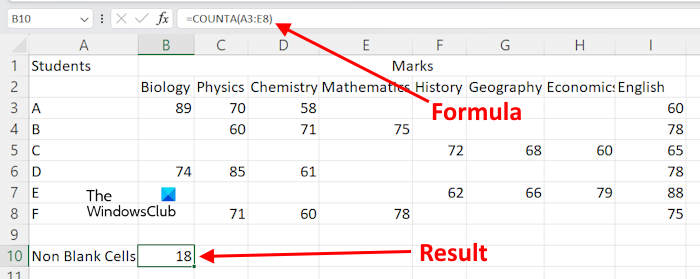You can also use your mouse cursor to select the cell range instead of typing the cell address manually. After typing the formula, hit Enter, and Excel will show you the result.

Suppose you want to count nonblank cells in Excel for different cell ranges. In this case, you have to use the formula in the following format.

`=COUNTA(cell range 1, cell range 2, ....)`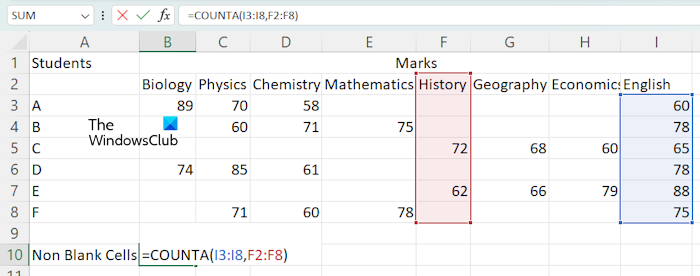Let’s use the above-mentioned formula to count nonblank cells in different cell ranges, like I3:I8 and F2:F8. In this case, the formula will become:

`=COUNTA(I3:I8,F2:F8)`

Hit Enter when you are done.

#### 2] Using the COUNTIF function to count nonblank cells

You can also use the COUNTIF function to count nonblank cells in Microsoft Excel. If you want to use this formula, you have to use the following format:

`=COUNTIF(cell range,"<>")`

Let’s use the above formula. I want to count nonblank cells in the cell range F2:I8, then the above formula will become:

`=COUNTIF(F2:I8,"<>")`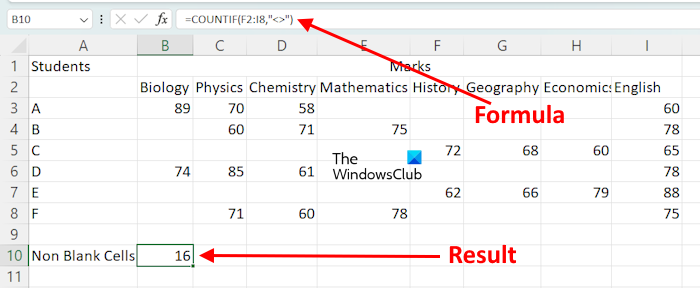In the above formula, we are using COUNTIF to count the cells within the given range, the value of which is not equal to nothing. In simple words, the cells that contain a character are counted.

If you want to use the COUNTIF function to count nonblank cells for different cell ranges, you have to use it with the SUM function. Here is the format of the formula:

`=SUM((COUNTIF(cell range,"<>"),COUNTIF(cell range,"<>"),...)`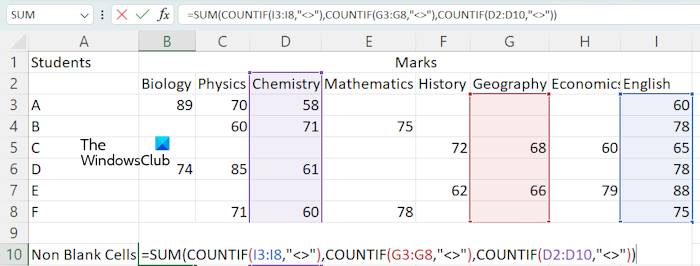For example, if I want to count nonblank cells by using the COUNTIF function for the cell ranges I3:I8, G3:G8, and D2:D10, then I will use the formula:

`=SUM(COUNTIF(I3:I8,"<>"),COUNTIF(G3:G8,"<>"),COUNTIF(D2:D10,"<>"))`

When you are done, hit Enter, and Excel will display the result.

#### 3] Count nonblank cells in Excel by using the Find and Replace feature

The Find and Replace feature in Excel is used to find something in your worksheet and replace it with something. You can use it to count nonblank cells. Here is how to use it.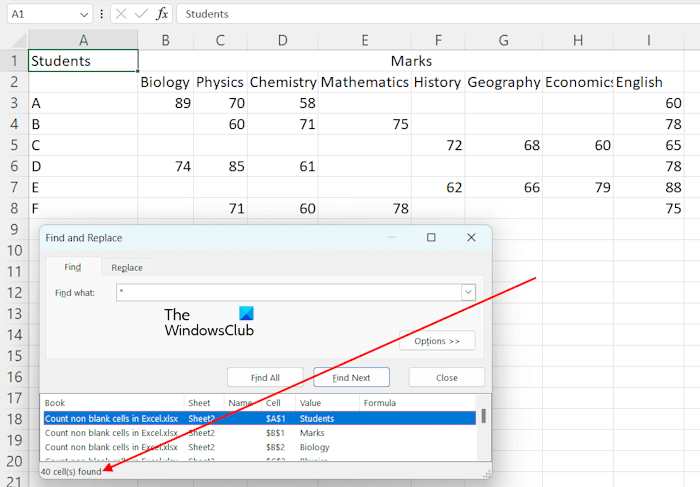In Excel, the * is used to find all the cells that are not empty. We will use it to count all nonblank cells. Press the Ctrl + F keys to open the Find and Replace window. Type * in the Find what field under the Find tab. Now, click Find All. After that, Excel will show you the total number of nonblank cells along with their addresses and values.

If you want to count nonblank cells within a specified range, you can also do so with the help of the Find and Replace feature. In this case, select the range of cells, then open the Find and Replace window by pressing the Ctrl + F keys. Now, click Find All.

That’s it. I hope this helps.

### Why is COUNTA not working?

If COUNTA is not working and showing you the wrong results. There may be some cells that look empty but actually contain some value, like space. COUNTA counts all the cells that are not empty. Hence, in this case, Excel is displaying the wrong result.

### What is the difference between COUNT Blank and COUNTA?

The COUNTBLANK function is used to count blank cells in Excel. Whereas, the COUNTA function is used to count the cells that are not blank.